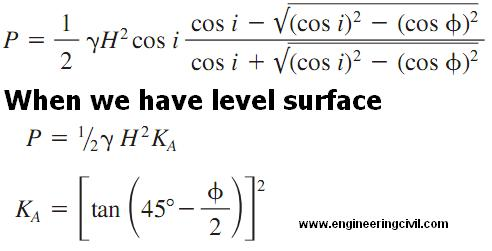Awarded as the best online publication by CIDC

# Soil Engineering

Lateral Pressure From Surcharge

To counter any effect of a surcharge on retaining wall on a cohesionless soil or an unsaturated cohesive soil we need to apply a uniform horizontal load of magnitude KAp. This load is applied over the entire wall height.

In case of saturated cohesive soil, the entire surcharge value acts on the entire wall height. This is considered to be a uniform load acting horizontally.

What is the Water Pressure Retained by Retaining Walls?

We know that water exerts a pressure on the wall and this thrust is calculated by using the following formula

P = ½Yo H2
where H = height of water above bottom of wall, ft (m)
Yo= unit weight of water.

Unit of water is 62.4 lb/ft3 1001g/m for freshwater and 64 lb/ft3 or 1026.7 kg/m3 for saltwater.

The thrust applied by water is considered to be acting at a distance of H/3 from the bottom of the retaining wall. The pressure distribution is triangular and has the maximum pressure of 2P/H at the bottom of the wall.

What is Lateral Pressures In Cohesionless Soils?

The lateral pressure in cohesion less soils is given by following formula:-

Following terms are used in the formula given below

Y=unit weight of soil, lb/ft3 or kg/m3
P=total thrust of soil, lb/linear ft (kg/m) of wall
H= total height of wall, ft (m)
KA= coefficient of active pressureWhat is the Lateral Pressure In Soils?

We know that The Rankine theory of lateral earth pressures is the most widely used theory to find the lateral pressure which is acting on retaining walls. But this theory has some assumptions which we need to know before hand.

Assumptions in the Rankine Theory of Lateral Earth Pressures
1) The pressure on the back of a vertical wall is the same as the pressure that would exist on a vertical plane in an infinite soil mass.
2) Their is no Friction between the wall and the soil.

What is the Lateral Pressure of Cohesive Soils?

P=1/2YH2KA-2cH(KA)1/2
where
Y=unit weight of soil, lb/ft3 or kg/m3
P=total thrust of soil, lb / linear ft (kg/m) of wall
H= total height of wall, ft (m)
KA= coefficient of active pressure
c= cohesion, lb/ft2or kPa

Physical Properties Of Soils

The soil properties and parameters can be broadly classified as
1)Physical
2)Engineering
3)Index

Physical soil properties include specific gravity, density, particle size and distribution and water content.
The water content represented by w of a soil sample is defined as the weight of free water in the sample expressed as a percentage of its dry weight.

The degree of saturation S is defined as the ratio of the volume of free water to its total volume of voids Vv

n=Vv/V
where n is the Porosity defined as the ratio of void volume to the total volume V of soil.